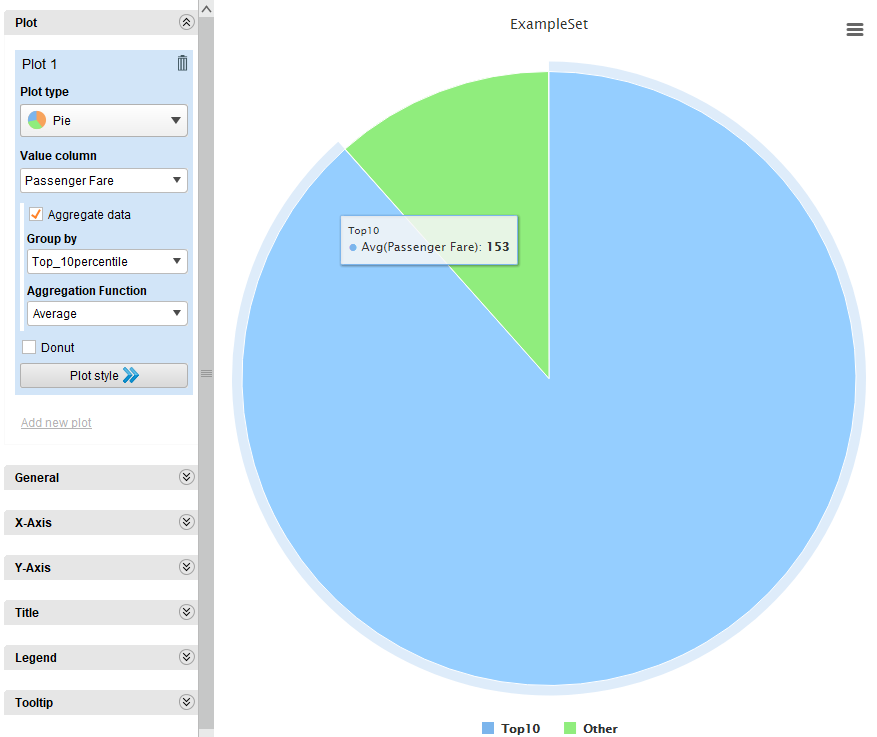# Grouping data and creating a pie chart

Member Posts: 1Newbie
edited October 2020 in Help
Hello.

I have a question on how to group data and measure it against other data in the same set.
I would like to group the top 10 data and create a pie chart showing the total percentage of the top 10 data and the total percentage of all the others.
Basically, I wanted the pie chart to be divided into "top 10" and "others".
I really have no clue where to start.
I hope anyone could help.

Thank you so much.
Tagged:

• Administrator, Employee, RapidMiner Certified Analyst, RapidMiner Certified Expert, Member Posts: 363RM Data Scientist
Hi @noelle,

The pie chart will need a target for "top 10" or "others". I group the Titanic passenger fare into two categories: top 10 percentile or others. The pie char shows an example to view the average fare by group.```<?xml version="1.0" encoding="UTF-8"?><process version="9.7.002">
<context>
<input/>
<output/>
<macros/>
</context>
<operator activated="true" class="process" compatibility="9.7.002" expanded="true" name="Process">
<parameter key="logverbosity" value="init"/>
<parameter key="random_seed" value="2001"/>
<parameter key="send_mail" value="never"/>
<parameter key="process_duration_for_mail" value="1"/>
<parameter key="encoding" value="SYSTEM"/>
<process expanded="true">
<operator activated="true" class="retrieve" compatibility="9.7.002" expanded="true" height="68" name="Retrieve Titanic Training" width="90" x="45" y="34">
<parameter key="repository_entry" value="//Samples/data/Titanic Training"/>
</operator>
<operator activated="true" class="sort" compatibility="9.7.002" expanded="true" height="82" name="Sort" width="90" x="179" y="34">
<parameter key="attribute_name" value="Passenger Fare"/>
<parameter key="sorting_direction" value="decreasing"/>
</operator>
<operator activated="true" class="generate_id" compatibility="9.7.002" expanded="true" height="82" name="Generate ID" width="90" x="313" y="34">
<parameter key="create_nominal_ids" value="false"/>
<parameter key="offset" value="0"/>
</operator>
<operator activated="true" class="generate_attributes" compatibility="9.7.002" expanded="true" height="82" name="Generate Attributes" width="90" x="447" y="34">
<list key="function_descriptions">
<parameter key="Top_10percentile" value="if(id&lt;92,&quot;Top10&quot;,&quot;Other&quot;)"/>
</list>
<parameter key="keep_all" value="true"/>
<description align="center" color="transparent" colored="false" width="126">top10 percentile fare or others</description>
</operator>
<connect from_op="Retrieve Titanic Training" from_port="output" to_op="Sort" to_port="example set input"/>
<connect from_op="Sort" from_port="example set output" to_op="Generate ID" to_port="example set input"/>
<connect from_op="Generate ID" from_port="example set output" to_op="Generate Attributes" to_port="example set input"/>
<connect from_op="Generate Attributes" from_port="example set output" to_port="result 1"/>
<portSpacing port="source_input 1" spacing="0"/>
<portSpacing port="sink_result 1" spacing="0"/>
<portSpacing port="sink_result 2" spacing="0"/>
</process>
</operator>
</process>
```

Hope it helps.

YY